# 1st PUC Chemistry Model Question Paper 1 with Answers

Students can Download 1st PUC Chemistry Model Question Paper 1 with Answers, Karnataka 1st PUC Chemistry Model Question Papers with Answers helps you to revise the complete Karnataka State Board Syllabus and score more marks in your examinations.

## Karnataka 1st PUC Chemistry Model Question Paper 1 with Answers

Time: 3.15 Hours
Max Marks: 70

Instruction:

1. The questions paper has five parts A, B, C, D and E. All parts are compulsory.
2. Write balanced chemical equations and draw labeled diagram wherever allowed.
3. Use log tables and simple calculations f necessary (use of scientific calculations is not allowed).

Part – A

I. Answer ALL of the following (each question carries one mark): ( 10 × 1 = 10 )

Question 1.
What is limiting reagent?
In a reaction, the reactant which reacts completely and present in small amount than the other reactant is called limiting reagent.

Question 2.
How does viscosity varies with temperature.
Viscosity ∝ $$\frac{1}{\text { Temperature }}$$

Question 3.
Give an example of acidic buffer.
CH3COOH + CH3COONaQuestion 4.
Which of the following ion is iso electronic with Na+
(a) Ca2+ (b) Mg2+ (c) Zn2+
Mg2+

Question 5.
Define reduction based on electron transfer.
Gain of electron by an atom/ion/molecule is called reduction.

Question 6.
Write the molecular formula of plaster of Paris.
2CaSO4.2H2O

Question 7.
The maximum co-valency of boron is 4. Why?
It has only four orbitals is its valence shell. (B = 5 = 1s22s2px2py2pz)

Question 8.
What type of hybridized carbon atom present in diamond?
sp3

Question 9.
Write the IUPAC name of CH2=CH-CO-CH3
But-3-ene-2-one.Question 10.
Name the catalyst used in Friedel-Craft’s reaction.
Anhydrons aluminium chloride (Anhyd AlCl3).

Part – B

II. Answer any FIVE of the following questions carrying TWO marks: ( 5 x 2 = 10 )

Question 11.
Define molar volume. What is its value at STP?
Volume occupied by one mole of a gas at STP. 22400cm3 or 22.4 dm3

Question 12.
What is the compressibility factor? What is its value for ideal gas?
It is the ratio of pressure x volume and RT
i.e, Z = $$\frac{P V}{R T}$$ or Z = $$\frac{\mathrm{PV}}{\mathrm{nRT}}$$
For ideal gas, Z = 1

Question 13.
Write any two postulates of VSEPR theory.

1. Repulsion of electron pair is in the order BP – BP < BP – LP < LP – LP.
2. The geometry of the molecule depends on the no of BP and LP electrons around the central atom.

Question 14.
Write the chemical reactions for the formation off
(a) Bleaching powder from milk of lime.
(b) Quick lime form lime stone.
(a) 2Ca(OH)2 + 2Cl2 → CaCl2 + Ca(OCl)2 + 2H2O
(b)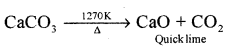Question 15.
How many carbon atoms are present in Buckminsterfullerene? What is the hybrization of each carbon atom in it?

1. C60
2. Sp2 hybridised

Question 16.
Explain ozonolysis of propene.
Propene reacts with O3 in CCl4 or CHCl3 at low temperature gives ozonides followed by reduction with Zn dust and water gives Ethanal and methanol.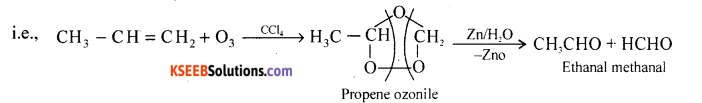Question 17.
Write the resonance structures and resonance hybrid structure of benzene.Question 18.
Name any two gases responsible for Green House effect.
CO2 and methane or H2O vapours or CFC.

Part – C

III. Answer any FIVE of the following questions carrying THREE marks: ( 5 x 3 = 15 )

Question 19.
(i) What is electron gain enthalpy? How it vary across a period in the periodic table?
(ii) Name the most electronegative element in the periodic table.
(i) The amount of energy released during the addition of electron (s) to neutral gaseous isolated atom to form anion.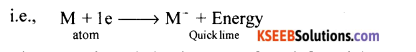(ii) Electron gain enthalpy increases from left to right across periods.

Question 20.
Give the conditions for the linear combination of atomic orbitals to form molecular orbitals.

1. Atomic orbitals energy should be equivalent.
2. Atomic orbitals should have same symmetry.
3. The percentage of overlaping should be large.

Question 21.
(a) What is hydrogen bonding? What type of hydrogen bonding is present in o-nitrophenol?
The force of attraction that binds hydrogen atom to electronegative atoms like oxygen, nitrogen and fluorine is called H bonding. In orthonitrophenol – Intramolecular hydrogen bonding present.

(b) Mention the shape of ammonia molecule based on VSEPR theory.
Pyramidal shape.

Question 22.
(a) Write any two drawbacks of the octet theory.

1. Hydrogen not obeys octet rule in its molecules but completes duplet.
2. Presence of less than 8 electrons in some compounds. Example: BeCl2, BF3, AlCl3 etc.
3. Presence of more than 8 electrons on the central atoms in certain compounds.
Example: PCl5, SF6, IF7, H2SO4

(b) Write the Lewis dot structure of CO molecule.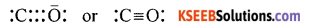Question 23.
Balance the following redox reaction by half reaction method.
Fe2+(aq) + Cr2O72- (aq) → Fe3+ (aq) + Cr3+(aq) : Acidic medium.
Step-1: Write the oxidation number of all the elementsStep-2: Oxidation and reduction half reactionsStep-3: 6Fe2+ → 6Fe3+ + 6e (change in oxidation number of Cr is +3)

Step-4: Balance the oxygen atoms and H+ ion
6Fe2+ + Cr2O7 + 14H+ → 6Fe3+ + 2Cr3+ + 7H2O

Question 24.
(i) Calculate the strength of 20 volume of solution of hydrogen peroxide.
(ii) What is the molecular formula of heavy water?

1. 20 volume H2O2 means, 2 litre of 20V of H2O2 gives 20 litres of oxygen at STP (NTP).
2. Consider the reaction:∴ 20 litre of 02 at STP will be obtained from H2O2
= $$\frac{68 \times 20}{22.4}$$  = 60.7g
∴ Strength of 20 volume of H2O2 solution = 60.7g/c

(ii) D2O.Question 25.
Give any one biological importance of each.
(a) Sodium (b) Magnesium (c) Calcium
(a) (i) Helps in the transmission of nerve signals.
(ii) Maintaining water balance across the cell membrane.

(b) (i) Magnesium helps in photosynthesis.
(ii) Act as catalyst for phosphate linkages in ATP.

(c) (i) In blood clotting, to maintain regular heart beating.

Question 26.
Give the differences between diamond and graphite with respect to structure, hybridization and electrical conductivity.
Graphite  :

1. Hexagonal layer structure.
2. Sp2 hybridised carbon atom.
3. Good conductor of electricity (due to the presence of unpaired electron on each C-atom.

Diamond :

1. Tetrahedral structure.
2. Sp3 hybidised carbon.
3. Bad conductor due to the absence of unpaired electron (s) on each carbon atom.

Part – D

IV. Answer any FIVE of the following questions carrying FIVE marks: ( 5 x 5 = 25 )

Question 27.
(a) N2 and H2 react with each other to produce ammonia according to the equation N2(g) + 3H2(g) → 2NH3(g)
(i) Calculate the mass of ammonia produced if 2.0 x 103 g of N2 reacts with 1.0 x 103 g of H2.
(ii) Will any of the two reactants remain unreacted?
(iii) If yes, which one and what would be its mass?

(b) State and illustrate law of multiple proportions.
(a) [28g of N2 (1 mole) reacts with 6g (3 mole) of H2
∴ 2.00 x 103 g of N2 react with H2 = $$\frac{6}{28}$$ x 2.00 x 103g = 428.57g
∴ N2 is a limiting reagent, H2 is excess reactant.

(i) 28g of N2 produces 34g of NH3
∴ 2.00 x 103 g of N2 produces NH3 = $$\frac{34 \times 2.00 \times 10^{3} \mathrm{g}}{28}$$ = 2428.57g.

(ii) H2 will remain unreacted (1.00 x 103 – 428.57).

(iii) Mass left unreacted = 1.00 x 103 – 428.57 = 571.43g.

(b) Two or more compound are formed from two elements, thus the masses of one of the element which combine with a fixed mass of the other element bear a simple ratio to one another.Here mass of C is fixed, combines with 16 and 32 g of oxygen. Hence the ratio 1:2 or 16:32.

Question 28.
(a) Mention the conclusion drawn from the observation of Rutherford’s a-ray scattering experiment.
(b) Write all the possible values of 1, m and w when n = 2.
(a) (i) Atom has large empty space
(ii) There should be a positive body present inside the atom.
(iii) The small positively changed body present within the atom was called nucleus.
(iv) The nucleus of the atom was heavier than cc-particles.

(b) When n = 2, l = 1 value
m = (2l + 1) ⇒ (2 x 1+1) = 3 values (+1, 0, -1)
S = + $$\frac{1}{2}$$ and $$\frac{1}{2}$$

Question 29.
(a) Calculate the energy associated with the first orbit of He+ (Z = 2, RH = 2.18 x 10-18J).
(b) What is a black body?
(c) Name the spectral region in which the Lyman series are present.
What is the colour of the first spectral line of the Balmer series.
(a) WKT = En = $$\frac{-2.18 \times 10^{-18} Z^{2}}{n^{2}}$$ J/atom
For He+, n = 1, Z = 2
∴E1 = $$\frac{-2.18 \times 10^{-18} \times 2^{2}}{1^{2}}$$ = -8.72 x 10-18

(b) Which is a perfect absorber and perfect emiter of energy.

(c) (i) ultra violet region/uv region
(ii) Red colour.Question 30.
(a) Using Dalton’s law of partial pressure derive an expression for partial pressure of a gas in terms of mole fraction.
(b) With a suitable example explain dipole-dipole forces.
(a) Consider a non-reacting gases A, B, C etc
Then apply PV = nRT(b) The attraction between polar molecules is called dipole-dipole interaction.
eg: H+_______Cl_______H+_______Clδ-
Here hydrogen atom acuires partial +ve change and Cl atom acuires partial -ve change. .’. they are attracted each other.

Question 31.
(a) Calculate the lattice enthalpy of sodium chloride using Born-Haber cycle.
(b) Give one example each for intensive and extensive property.
(a) Lattice enthalpy of NaCl:

(i). Sublimation of Na(s) to Na(g)
i.e., Na(s) → Na(g) ∆subH = ∆H

(ii) Dissociatiomn of Cl molecule to atom chlorine
$$\frac{1}{2}$$ Cl2 → Cl(g), ∆H = 1/2 ∆dissH

(iii) Ionization of Na(g) to Na+(g)
Na(g) → Na+(g) + le ∆iH = IE

(iv) Conversion of Cl(g) to Cl ion
C(g) + le → Cl, ∆egH = EA

(v) Combining of Na+(g) and Cl(g) to form 1 mole of crystalline sodium chloride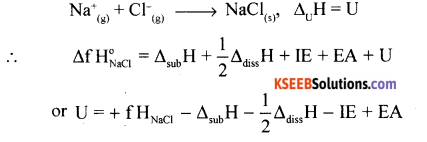(b) Intensive property = Pressure/density/BP/freezing point/mole fraction/molarity
Extensive property = Mass/volume/enthalpy/entropy.

Question 32.
(a) What is entropy? How does entropy changes in a spontaneous process?
(b) The molar enthalpy of combustion of benzene is -3264.6 kJ mol-1.
Calculate the amount of heat energy liberated when 39 grams of benzene is burnt in excess of air. [Given: Molar mass of benzene is 78 g mol-1].
(c) What is free expansion?
Measure of disordemess of the system. Entropy is +ve for spontaneous process.

(b) 78g/mol of benzene on combustion gives = 3264.6 kJ
39g of benzene on combustion = $$\frac{39 \times-3264.6 \mathrm{kJ} / \mathrm{mol}}{78 \mathrm{g}}$$ = 1632.3 kJ/mol
Heat of combustion of 39 g of benzene = -1632.3 kJ/mol.

(c)  The expansion of ideal gas against vaccum, the irreversible expansion is called free expansion.

Question 33.
(a) The value for the Kc. for the reaction 2A ⇌ B + C is 2 x 10-3. At a given time the
composition of reaction mixture is [A] = [B] = [C] = 3 x 10-4. In which direction the reaction will proceed?
Write the conjugate acid and base of water.
The equilibrium constant for the reaction H2(g) + I2(g) ⇌ 2HI(g) is K.
What is the equilibrium constant for the reaction HI(g) ⇌1/2 H2(g) + 1/2 I(g)?
(a) Solution: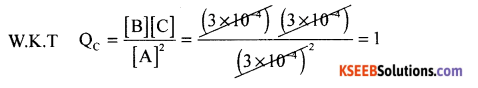Here Qc > Kc. Hence the reaction will proceed is the backward direction, (b) Conjugate acid of water = H3O+
Conjugate base of water = OH
(c) K = $$\frac{1}{\sqrt{\mathrm{K}}^{\mathrm{I}}}$$Question 34.
(a) The pKa of acetic acid and pKb of ammonium hydroxide are 4.76 and 4.75 respectively. Calculate the pH of ammonium acetate solution.
(b) State Le Chatelier’s principle? What is the effect of pressure on the following equilibria. CO(g) + 3H2(g) ⇌ CH4(g) + H2O(g)
(c) Write the solubility product expression for BaSO4
(a) Solution:
W.K.T
PH = 7 + $$\frac{1}{2}$$ [ PKa – PKb]
= 7 + $$\frac{1}{2}$$ [4.76 – 4.751 = 7 + 0.01 x $$\frac{1}{2}$$ = 7 + 0.005 = 7.005
PH of ammonium acetate = 7.005

(b) Statement:
When a system in equillibrium is subjected to a change in concentration, temperature or pressure, the equillibrium shift in a direction to nullify that change applied.
For CO(g) + 3H2(g) = CH4(g)+ H2O(g), [∆RHS = 2, LHS = 4]
∴ On increasing the pressure, the equillibrium shifts in forward direction to reduce the pressure.

(c) Ksp = [B02+ ] [ $$\bar{S} O_{4}^{–}$$ ] .

Part – E

V. Answer any TWO of the following questions carrying FIVE marks: ( 2 x 5 = 10 )

Question 35.
(a) 0.6723g of an organic compound gave on combustion 1.530g of carbon dioxide and 0.625 g of water. Find the percentage of carbon and hydrogen in the compound.
(b) Explain why an organic liquid vaporizes at room temperature below its boiling point in its steam distillation.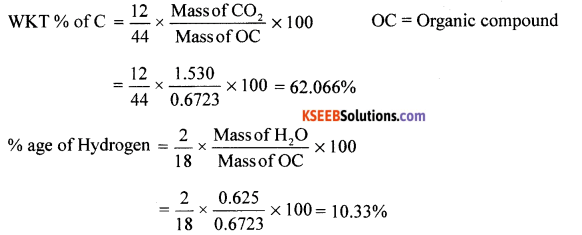(b) At same temperature, on reducing the pressure on the liquid, boils at lower temperature without decomposition. Therefore a pressure of 10-20 mm Hg reduces the BP by 100 degrees. Hence liquid boils without decomposition.

Question 36.
(a) Explain Kjeldahl’s method for the estimation of nitrogen in the organic compound? (b) Define (i) Resonance stabilization energy. (ii) Theoretical plate.
(a) Principle: A known mass of the organic compound is heated with cone H2SO4 in presence of potassium sulphate and little copper sulphate or mercury in a long-necked flask called Kjeldahl’s flask (K2SO4 rises the BP of H2SO4). Nitrogen present in the organic compound converted to ammonium sulphate, it in boiled with excess of NaOH solution to liberate NH3 gas which is then absorbed in a known excess of standard H2SO4 or HCl.
Calculation:(b) (i) Resonance stabilization energy: The difference in internal energy of the resonance hybrid and that of most stable canonical form or structure.
(ii) Theoritical plate: A separation process of hypothetical zone or stage in which two phases such as liquid phase and vapour phases of substance establish an equillibrium with each other.Question 37.
(a) Explain the mechanism of Friedel-craft’s alkylation of benzene to get toluene.
(b) Complete the following reaction:What happens to the colour of KMnO4 during the reaction ‘a’.
(a) Mechanism of Fried-Crafts Alkylation:

Step-1: Generation of electrophile
R – Cl + AlCl3 → R+ + AlCl3 OR
CH3Cl + AlCl4 → $$\overrightarrow{\mathrm{C}}$$ H3 + AlCl4

Step-2: Attack of $$\overrightarrow{\mathrm{C}}$$ H3 to Benzene ring and formation of carbocationStep – 3:
Loss of proton ( H+ )(b) (i)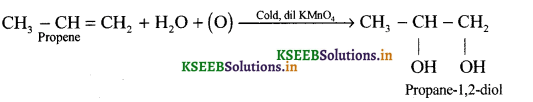(ii) Pink colour of KMnO4 is discharged.

error: Content is protected !!